AnyLogic

# Defining animation scale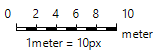Quite often we cannot animate large objects (warehouses, railway stations, etc.) in their actual size. That is why when animating such objects we reduce their sizes several times, i.e. scale the animation of the objects being modeled.

With the special element Scale you can set the scale of the animation, i.e. the ratio of the object’s animation to the actual size of the object. Actually the scale is set as a ratio of pixels of the animation to the physical units of length.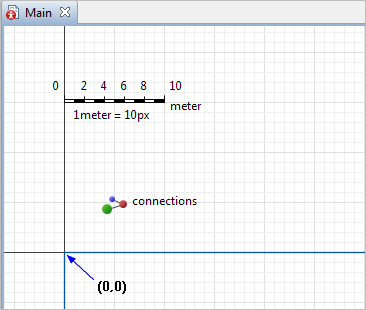Scale

You can see that there is always a chosen unit of length to pixel correspondence notice under the scale element for your convenience.

The scale element does not belong to any level.

There are two alternative methods to set the animation scale through use of the element Scale:

• Taking into consideration the dimensions of the animation figure. This mode is used when the scale of the animation is associated with the object’s real size.
• Just specifying the correspondence between physical units of length and pixels of model’s animation. This mode is used when you select the optimal scale on your own.
You define scale once for all the shapes on the agent diagram, so there can be only one ruler on the agent diagram. However, different agents may have different scales.

You can use the Properties view to set the required scale, or drag its end to set the number of pixels that should correspond to one unit of length. There are two modes that you can use to set up the scale. In the Properties view of the scale, you will find the property Scale is: Defined graphically or Specified explicitly:

• The Defined graphically option is the default choice for the scale. And also by default, the Ruler length corresponds to: 10 meters. It means that if you drag the ruler end in the graphical editor, its length will remain conventional 10 meters, and the number of pixels will be divided by ten to define how many pixels are in one meter. So, to change the scale, drag its square handle or change the Length, pixels property. Since the ruler length itself does not change (it remains 10 meters) the scale will change when the number of pixels it covers changes.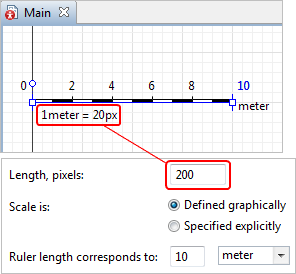• Another way is for the scale to be Specified explicitly. In this case, to change the scale, specify the number of pixels that correspond to one unit of length in the Scale field located below. Choose the required unit of length from the drop-down list on the right-hand side. Now, even if you change the Length, pixels property here, the scale itself will remain the same.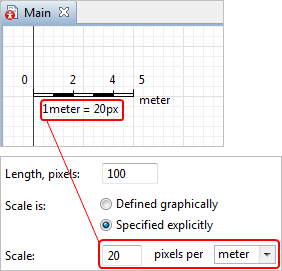To set the scale of agent animation according to its real size

Let us define the scale, for instance, for a train car animation in the model.

1. Move the diagram down and you will find the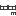Scale element located above X-axis on the agent’s diagram.
2. Move the scale ruler so that it coincides exactly with the animation figure length. First, position the scale along the figure. To move with pixel precision, hold down Alt key while dragging the element with the mouse. We can also recommend you also to zoom the graphical editor diagram using the Zoom In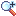toolbar button.
3. Match the end points of the ruler with the figure length. Stretch the scale ruler by dragging its end with the mouse. We again recommend holding down the Alt key while dragging.
4. Now set the required scale. In this particular case the train car real length corresponds to 27 meters. Go to the ruler Properties view. Leave the Scale: Defined graphically option selected. Specify 27 meters in the Ruler length corresponds to field below.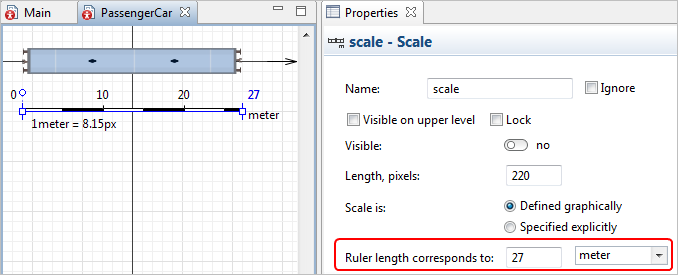5. You can see that the dimensions are the same now on both dimension lines. Now you can easily set object lengths and distances for a given type of agent, and AnyLogic will use the scale that you have set here.
6. If we run the model now, we will get realistic size right away: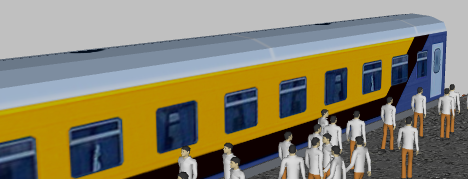## Properties

General

Visible on upper agent — If selected, the scale is also visible on the upper agent where this agent lives.

Lock — If selected, the scale is locked. Locked shapes do not react to mouse clicks — it is impossible to select them in the graphical editor until you unlock them. It is frequently needed when you want to prevent editing this shape while drawing other shapes near it.

Visible — Here you specify whether the shape is visible on the animation at the model runtime, or not. Using the control, choose yes or no.

Length, pixels — The length of the shape on an agent’s diagram, in pixels.

Scale is — Here you can choose how this element defines the scale of your model’s animation.

• Defined graphically — Use this mode if you know the real size of the objects that the animation figures represent.
• Specified explicitly — Use this mode if you know the exact scale for this agent’s animation.

AnyLogic supports both popular measurements: metric system (units of length: cm, m, km) and English (inches, feet, miles).

Position

X — X-coordinate of the Scale element.

Y — Y-coordinate of the Scale element.

Rotation — The rotation angle of the Scale element.